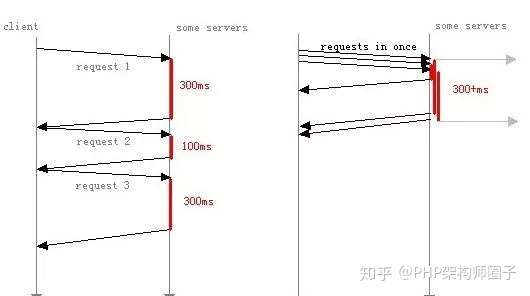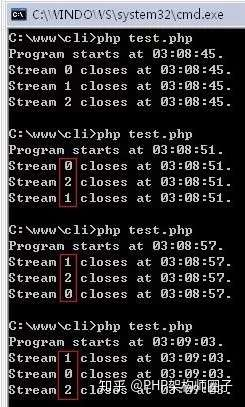# 非阻塞模式与PHP多进程

2020/10/14 11:12<?php
echo "Program starts at ". date('h:i:s') . "./n";
$timeout = 3;$sockets = array(); //socket句柄数组
//一次发起多个请求
$delay = 0; while ($delay++ < 3)
{
$sh = stream_socket_client("localhost:80",$errno, $errstr,$timeout,
STREAM_CLIENT_ASYNC_CONNECT|STREAM_CLIENT_CONNECT);
/* 这里需要稍微延迟一下，否则下面fwrite中的socket句柄不一定能真正使用
这里应该是PHP的一处bug，查了一下，官方bug早在08年就有人提交了
我的5.2.8中尚未解决，不知最新的5.3中是否修正
*/
usleep(10);
if ($sh) {$sockets[] = $sh;$http_header = "GET /test.php?n={$delay} HTTP/1.0/r/n";$http_header .= "Host: localhost/r/n";
$http_header .= "Accept: */*/r/n";$http_header .= "Accept-Charset: */r/n";
$http_header .= "/r/n"; fwrite($sh, $http_header); } else { echo "Stream failed to open correctly./n"; } } //非阻塞模式来接收响应$result = array();
$read_block_size = 8192; while (count($sockets))
{
$read =$sockets;
$n = stream_select($read, $w=null,$e=null, $timeout); //if ($n > 0) //据说stream_select返回值不总是可信任的
if (count($read)) { /* stream_select generally shuffles$read, so we need to
compute from which socket(s) we're reading. */
foreach ($read as$r)
{
$id = array_search($r, $sockets);$data = fread($r,$read_block_size);
if (strlen($data) == 0) { echo "Stream {$id} closes at " . date('h:i:s') . "./n";
fclose($r); unset($sockets[$id]); } else { if (!isset($result[$id]))$result[$id] = '';$result[$id] .=$data;
}
}
} else {
echo "Time-out!/n";
break;
}
}
//print_r($result);  几点说明： 1、使用stream_socket_client函数链接请求服务器和端口（简便起见这里使用同一地址localhost）。这里不受限于http协议，可广泛用于所有TCP/IP协议。详细内容请参考手册。 2、这里链接成功后通过发送各自http头信息来获取不同响应（这里使用网站根目录下的test.php做服务端）。 3、发送header前需要个微小的延迟，代码中已经做了注释。 CLI模式运行结果：多运行几次会发现，三次请求结束顺序是无序的。该demo太过简单导致整个过程一秒内已完成，但可以针对三次不同请求做相应延迟，来看出非阻塞时时间复用的效果。 下面再大概说下本地并发与PHP多进程编程与实例 本地并发只能通过语言自己的特性在程序本身实现多任务效果，一般来说现在的语言会通过多线程或多进程的方式来实现。由于PHP不支持多线程，目前只能采用多进程方式，让操作系统来帮助实现本地并发。 至于代码实现，可以通过pcntl扩展（封装fork等进程控制函数，和C语言中使用非常相似，windows下不可用）、 proc_openpopen等方式，方法不止一种， 场景：日常任务中，有时需要通过php脚本执行一些日志分析，队列处理等任务，当数据量比较大时，可以使用多进程来处理。 准备：php多进程需要pcntl，posix扩展支持，可以通过 php - m 查看，没安装的话需要重新编译php，加上参数--enable-pcntl，posix一般默认会有。 创建子进程的函数fork pcntl_fork — 在当前进程当前位置产生分支（子进程）。译注：fork是创建了一个子进程，父进程和子进程 都从fork的位置开始向下继续执行，不同的是父进程执行过程中，得到的fork返回值为子进程号，而子进程得到的是0。 一个fork子进程的基础示例： <?php$pid = pcntl_fork();//父进程和子进程都会执行下面代码
if ($pid == -1) { //错误处理：创建子进程失败时返回-1. die('could not fork'); } else if ($pid) {
//父进程会得到子进程号，所以这里是父进程执行的逻辑
pcntl_wait($status); //等待子进程中断，防止子进程成为僵尸进程。 } else { //子进程得到的$pid为0, 所以这里是子进程执行的逻辑。
}


split的用法比较简单，可以man split查看下手册。

split -l 25000 -d file.log prefix_name


-l是按照行分割，-d是分割后的文件名按照数字，-a是分割后的文件个数位数（默认是2，做多就是99个；比如超过100个，-a可以写3）。自己尝试分割一下就知道了。

<?php
shell_exec('split -l 25000 -d file.log prefix_name');
// 3个子进程处理任务
for ($i = 0;$i < 3; $i++){$pid = pcntl_fork();
if ($pid == -1) { die("could not fork"); } elseif ($pid) {
echo "I'm the Parent $i\n"; } else {// 子进程处理$content = file_get_contents("prefix_name0".$i); // 业务处理 begin // 业务处理 end exit; // 一定要注意退出子进程,否则pcntl_fork() 会被子进程再fork,带来处理上的影响。 } }// 等待子进程执行结束 while (pcntl_waitpid(0,$status) != -1) {
$status = pcntl_wexitstatus($status);
echo "Child \$status completed\n";}

0
0 收藏

### 作者的其它热门文章0 评论
0 收藏
0